EMI Suppression Filters (EMC and Noise Suppression)Noise Suppression Products/EMI Suppression Filters

Noise Suppression Basic Course Section 1
Chapter 1
Chapter 2
Chapter 3
Chapter 4
Chapter 5
Chapter 6
Chapter 6

EMI suppression filters

6-1. Introduction

There are two basic methods used to suppress noise: filters and shields. These have a mutually supportive relationship; although both are generally needed, either may be simplified if there is little noise. Shields are generally made of metal. Since they must cover the entire electronic device they tend to be large. This means that they also tend to increase the cost and weight of the device. Filters are electronic components, and making skillful use of them can allow you to simplify shields, and can help reduce the cost and weight of electronic devices.
This chapter gives a brief overview of filter operating principles and explains a typical circuit configuration in order to provide a good base of knowledge for making skillful use of filters. Filter circuits used to eliminate electromagnetic noise are typically called EMI suppression filters, but they will be referred to simply as "filters" here.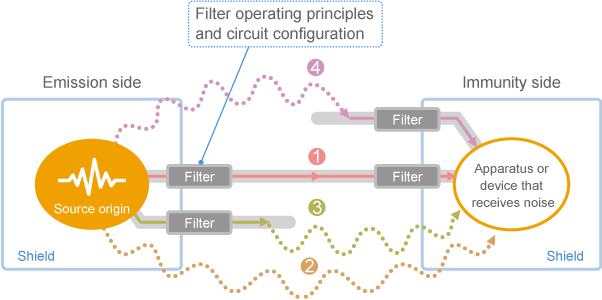Fig. 1 Topics covered in Chapter 6

6-2.　EMI suppression filters

6-2-1.　Operation of EMI suppression filters

Installing a filter on the noise transmission path separates components required for the circuit to operate (represented as "signal" in Fig. 1) from noise. The filter then removes only the noise.
In order to separate signals from noise, there needs to be some standard to separate them. Differences in frequency distribution are normally used for this purpose (as shown in Fig. 1 (b) ). However, there are also cases where differences in transmission mode or voltage are used. Table 1 lists the information that typical filters use to perform this function.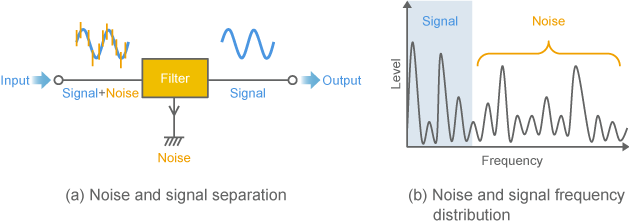Fig. 1 Filter operation
Type Target noise Information used to separate noise Difference in signal/noise Implementation method Major applications
EMI suppression filter Radio noise in general Deviation in frequency distribution Signal Low frequency Low-pass filter Electric circuits in general
Noise High frequency
Common mode choke coil Common mode noise Difference in transmission mode Signal Normal Coupling coil Power supplies (AC/DC)
Differential signal
Noise Common
Surge absorber High voltage surges Difference in voltage Signal Low voltage Non-linear resistance
Discharge gap, etc.
Electronic devices in general
(surge entry point)
Noise High voltage
Table 1 Signal and noise separation

6-2-2. Four filters arranged by frequency

As shown in Fig. 2, there are four major types of filters that separate noise by frequency. It is often difficult to narrow down target noise frequencies in advance, so low-pass filters are often used as the EMI suppression filter.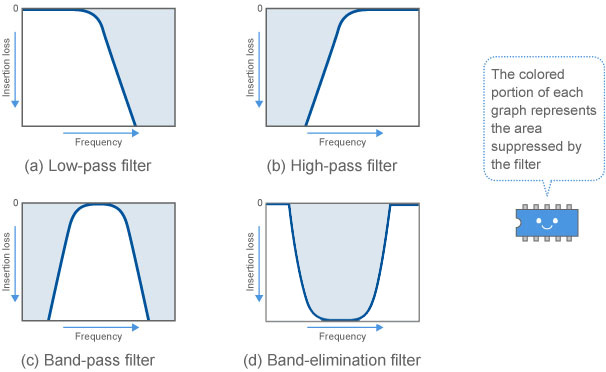Fig. 2 Major filter frequency characteristics

6-2-3. How to measure filter effectiveness

(1) Insertion loss

The noise reduction effect of a filter is represented by insertion loss. As shown in Fig. 3, a filter was inserted into a circuit connecting a 50 ohms signal source with a load, and then the change in voltage on the load side (B to C) was measured. This is normally expressed in dB.

(2) dB

dB (decibel) is a unit used to express a ratio. As shown in Table 2, the ability to reduce noise to 1/10th is expressed as 20dB, while the ability to reduce noise to 1/100th is expressed as 40dB. Each time noise drops to 1/10th, the dB increases by 20 (for insertion loss).
Using dB is very convenient as it can express a large ratio through addition. The result of measuring noise is also normally expressed in dB. For example, 40dBµV represents a voltage where 1µV is 0 dB, and so it means 100µV.

(3) Numbers to keep in mind

When noise doubles, the dB changes by 6dB. When noise increases tenfold, the dB changes by 20dB. These two numbers are used very often, so it can be useful to keep them in mind.
Note however that these numbers are used when representing voltage. For power, a tenfold increase results in a change of 10dB. This is because power has a relationship proportional to voltage squared (P=V2/R).

(4) S-parameter substitution

When a filter can be regarded as a linear circuit, insertion loss can be substituted by the absolute value of the S-parameter transmission coefficient (S21 or S12) measured on a 50 ohms system. This is because a 50 ohms measurement system is connected before and after the filter, for both measurements. Whenever possible, we use the value measured with the S-parameter as the insertion loss.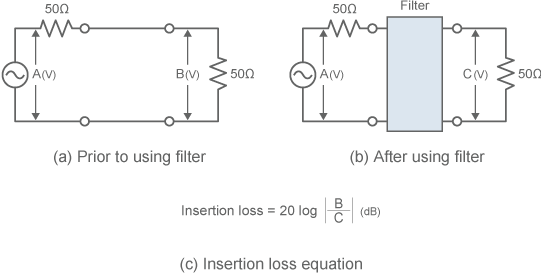Fig. 3 Insertion loss measurement circuit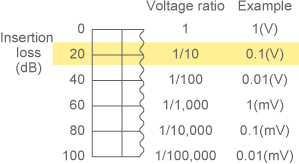Table 2 Insertion loss and noise reduction effect

EMI suppression filters" - Key points

• EMI suppression filters mainly use differences in noise and signal frequency in order to separate noise.
• Some EMI suppression filters use differences in noise and signal transmission mode or voltage in order to separate noise.
• Insertion loss is often used as a measurement of a noise filter's effectiveness at eliminating noise.